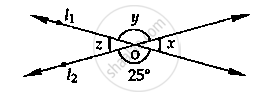# In the Given Fig, Find the Values of X, Y and Z. - Mathematics

In the given fig, find the values of x, y and z.#### Solution

From the given figure

∠y = 25°          [∴ Vertically opposite angles are equal]

Now

∠x + ∠y = 180°          [Linear pair of angles are x and y]

⇒∠x = 180° - 25°

⇒ ∠x = 155°

Also

∠z = ∠x = 155°          [Vertically opposite angle]

∠y = 25°

∠z = ∠z = 155°

Concept: Pairs of Angles
Is there an error in this question or solution?

#### APPEARS IN

RD Sharma Mathematics for Class 9
Chapter 10 Lines and Angles
Exercise 10.3 | Q 3 | Page 22